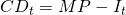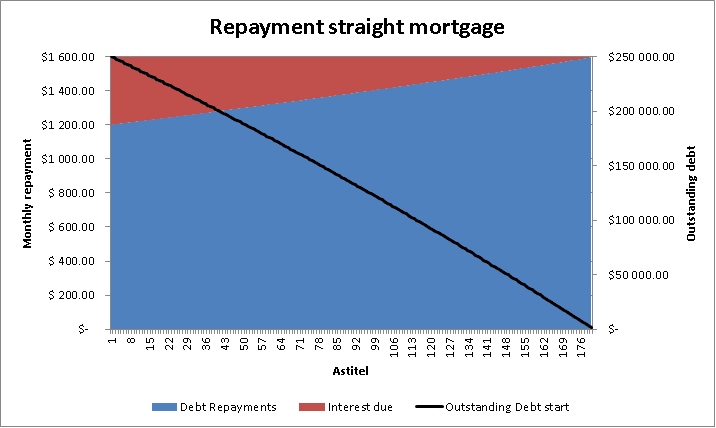# Annuity mortgage

An annuity is a type of mortgage for which periodically remain the same over the full life of the loan. It has the characteristic of having higher interest payments in the beginning which lower over time. In contrast the capital downpayments are initially lower, but increase as time passes by.

## Annuity mortgage formula

As a first step the annuity factor AF, needs to be calculated. This is done by applying the following formula in which you need to determine the interest rate i, the periodically repayments m in a given year and the maturity of the loan n in years: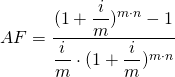As a second step the monthly payments can be calculated by dividing the total debt at the start of the mortgage B, by the annuity factor F.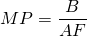The interest I that has to be paid over a monthly period is simply the outstanding debt at the beginning of the month multiplied by the annual interest rate r divided by the periodical payments pp, over a year e.g. 12.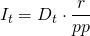The capital downpayment equals the monthly payment C minus the interest I due over the month.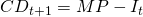The outstanding debt at the beginning of the next month equals the outstanding debt of the current month minus the capital downpayment: